SYLLABUS  Previous: 10.1 Glossary of keywords  Up: 10 APPENDIX  Next: 10.3 Final interactive evaluation

## 10.2 Notation and symbols

Slide : [ GLOSSARY: Bloomberg - Economist || DATA ]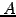value of a fixed amount of cash.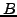barrier of an option, resistance / support level in a market.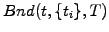value at time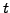of a bond paying coupons at times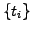up to the maturity date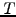.
C
plain vanilla call option.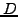fixed dividend per share.
E
earnings from an asset in the P/E ratio.
G
annual growth rate from an asset, similar to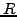.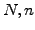numbers counting random walkers, days, intervals, etc.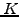strike price / rate of an stock / credit option, or swap rate.
P
price of an asset in the P/E ratio or plain vanilla put option.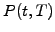value at timeof a discount bond with a maturity.continuously compounded interest rate.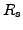simply compounded interest rate.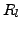discretely compounded (every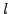years) interest rate.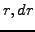spot rate and its increments for an interest rate.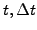time and time interval.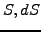spot price and its increment for a share.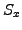Sharpe ratio for a portfolio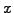.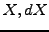unknown and its differential in general.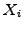pre-determined value of a bond coupon payed at time.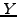annual yield measuring an exponential growth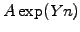.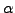CAPM parameter measuring the performance from arbitrage and costs.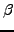CAPM parameter measuring the performance from taking risk.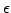relative error (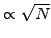in Monte-Carlo simulations.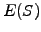expected value from a random variable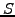.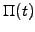value at timeof a portfolio.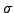volatility, standard deviation of expected returns.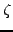random number

SYLLABUS  Previous: 10.1 Glossary of keywords  Up: 10 APPENDIX  Next: 10.3 Final interactive evaluation